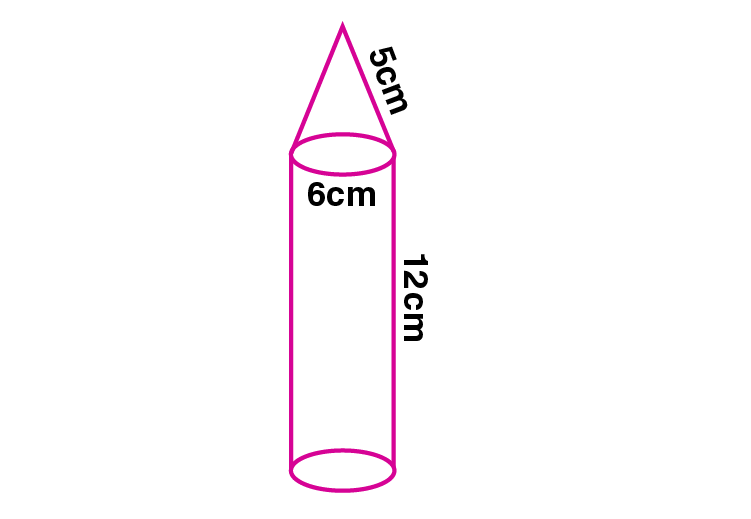Newbie

# A rocket is in the form of a right circular cylinder closed at the lower end and surmounted by a cone with the same radius as that of the cylinder. The diameter and the height of the cylinder are 6 cm and 12 cm respectively. If the slant height of the conical portion is 5 cm, find the total surface area and the volume of the rocket.

• -1

An Important Question of M.L Aggarwal book of class 10 Based on Mensuration Chapter for ICSE BOARD.
A rocket is in the form of a right circular cylinder closed at the lower end and surmounted by a cone with the same radius as that of the cylinder. The diameter and the height of the cylinder are given. If the slant height of the conical portion is given, find the total surface area and the volume of the rocket.
This is the Question Number 18, Exercise 17.4 of M.L Aggarwal.

Share

1.Given diameter of the cylinder = 6 cm

Radius of the cylinder, r = 6/2 = 3 cm

Height of the cylinder, H = 12 cm

Slant height of the cone, = 5 cm

Radius of the cone, r = 3 cm

Height of the cone, h = √(l2-r2)

h = √(52-32)

h = √(25-9)

h = √16

h = 4 cm

Total surface area of the rocket = curved surface area of cylinder +base area of cylinder+ curved surface area of cone

= 2rH+r2+rl

= r(2H+r+l)

= 3.14×3×(2×12+3+5)

= 3.14×3×(24+3+5)

= 3.14×3×32

= 301.44 cm2

Hence the Total surface area of the rocket is 301.44 cm2.

Volume of the rocket = Volume of the cone + volume of the cylinder

= (1/3)r2h +r2H

= r2((h/3)+H)

= 3.14×32×((4/3)+12)

= 3.14×9×((4+36)/3)

= 3.14×9×(40/3)

= 3.14×3×40

= 376.8 cm3

Hence the volume of the rocket is 376.8 cm3.

• 0